Author: Oscar Cronquist Article last updated on May 05, 2022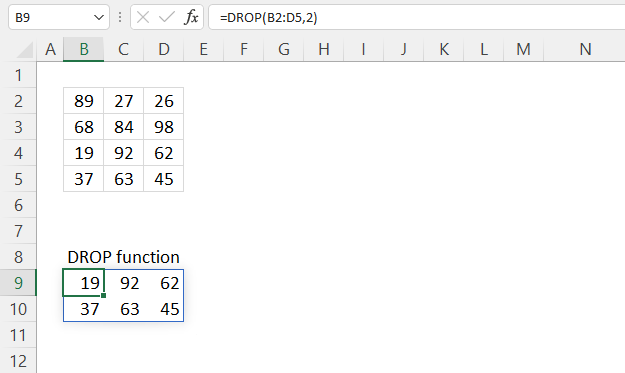The DROP function removes a given number of rows or columns from a 2D cell range or array.

Dynamic array formula in cell B9:

=DROP(B2:D5, 2)

The DROP function is available to Excel 365 users and is in the "Array manipulation" category.

## 1. DROP Function Syntax

DROP(array, rows, [columns])

## 2. DROP Function Arguments

 array Required. The source cell range or array. rows Required. The number of contiguous rows to remove, a negative number removes contiguous rows from the end. [columns] Optional. The number of contiguous columns to remove, a negative number removes contiguous rows from the end.

## 3. DROP Function example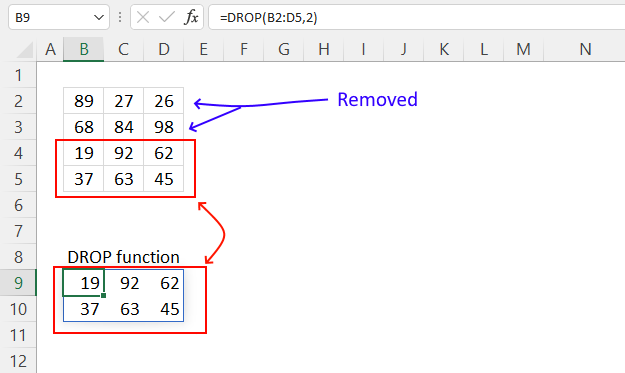The picture above shows how the DROP function removes the two first rows from cell range B2:D5.

Dynamic array formula in cell B9:

=DROP(B2:D5, 2)
Note! If any of the values in the source range change, the values in the DROP function output change as well.

### 3.1 Explaining formula

#### Step 1 - DROP function

DROP(array, rows, [columns])

array - B2:D5
rows - 2
[columns] -

#### Step 3 - Evaluate function

DROP(B2:D5, 2)

becomes

DROP({89, 27, 26;
68, 84, 98;
19, 92, 62;
37, 63, 45}, 2)

and returns

{19, 92, 62;
37, 63, 45}

## 4. DROP Function example - remove columns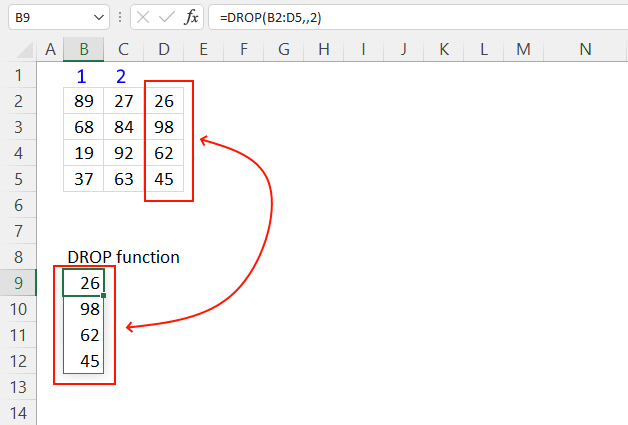The image above demonstrates how to remove columns using the DROP function.

Formula in cell B6:

=DROP(B2:D5, , 2)

The DROP function removes the two first columns from cell range B2:D5.

## 5. DROP Function example - return rows and columns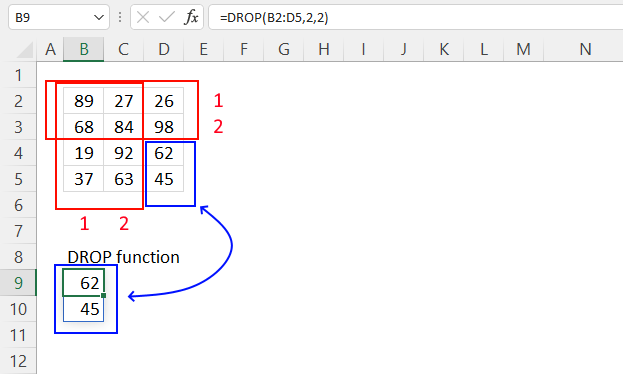The image above demonstrates how to remove both rows and columns using the DROP function.

Formula in cell B9:

=DROP(B2:D5, 2, 2)

DROP(array, rows, [columns])

## 6. DROP Function example - negative values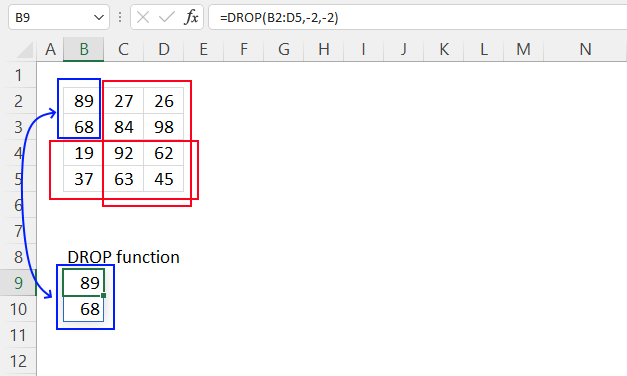The DROP function lets you use negative arguments, this means that the function removes rows/columns from the end.

Formula in cell B9:

=DROP(B2:D5, -2, -2)

DROP(array, rows, [columns])

## 7. DROP function error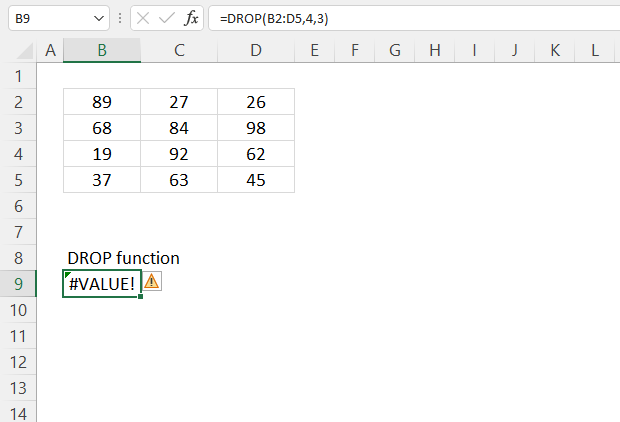The DROP function returns a #VALUE! error when no values are displayed.

## 8. DROP function - values in random order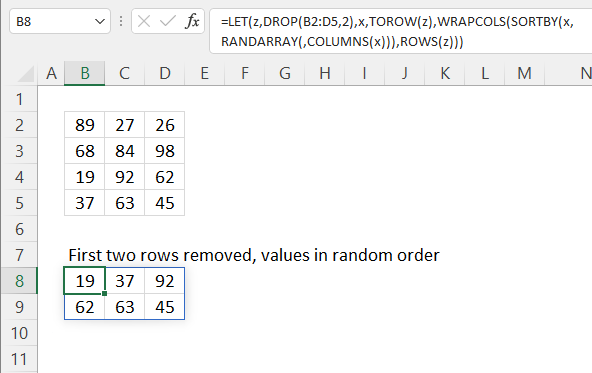The image above shows a formula that returns values in random order from the two first rows in a 2D cell range.

Dynamic array formula in cell B8:

=LET(z, DROP(B2:D5, 2), x, TOROW(z), WRAPCOLS(SORTBY(x, RANDARRAY(, COLUMNS(x))), ROWS(z)))

### 8.1 Explaining formula in cell B8

#### Step 1 - Remove two first rows

DROP(B2:D5, 2)

becomes

DROP({89, 27, 26;
68, 84, 98;
19, 92, 62;
37, 63, 45}, 2)

and returns

{19, 92, 62;
37, 63, 45}.

#### Step 2 - Rearrange values to a single row

The TOROW function rearranges values from a 2D cell range or array to a single row.

TOROW(array, [ignore], [scan_by_col])

TOROW(DROP(B2:D5,2))

becomes

TOROW({19, 92, 62;37, 63, 45})

and returns

{19, 92, 62, 37, 63, 45}.

#### Step 3 - Count cells

The COLUMNS function returns the number of columns in a given cell range or array.

COLUMNS(array)

COLUMNS(TOROW(DROP(B2:D5,2)))

becomes

COLUMNS({19, 92, 62, 37, 63, 45})

and returns 6.

#### Step 4 - Count rows

The ROWS function returns the number of rows in a given cell range or array.

ROWS (array)

ROWS(DROP(B2:D5,2))

becomes

ROWS({19, 92, 62;37, 63, 45})

and returns 2.

#### Step 5 - Create random decimal numbers

The RANDARRAY function returns a table of random numbers across rows and columns.

RANDARRAY([rows], [columns], [min], [max], [whole_number])

RANDARRAY(,COLUMNS(TOROW(DROP(B2:D5,2))))

becomes

RANDARRAY(,6)

and returns

{0.215398134613085, 0.390607168196479, ... ,0.83231474462401}.

#### Step 6 - Rearrange values in random order

The SORTBY function allows you to sort values from a cell range or array based on a corresponding cell range or array.

SORTBY(array, by_array1, [sort_order1], [by_array2, sort_order2],…)

SORTBY(TOROW(DROP(B2:D5,2)),RANDARRAY(,COLUMNS(DROP(B2:D5,2))))

becomes

SORTBY({19, 92, 62, 37, 63, 45}, {0.215398134613085, 0.390607168196479, ... ,0.83231474462401})

and returns

{19, 92, 62, 37, 63, 45}.

#### Step 7 - Rearrange values to the original array size

The WRAPCOLS function rearranges values from a single row to a 2D cell range based on a given number of values per column.

WRAPCOLS(SORTBY(TOROW(DROP(B2:D5,2)),RANDARRAY(,COLUMNS(TOROW(DROP(B2:D5,2))))),ROWS(DROP(B2:D5,2)))

becomes

WRAPCOLS({19, 92, 62, 37, 63, 45}, ROWS(DROP(B2:D5,2)))

becomes

WRAPCOLS({19, 92, 62, 37, 63, 45}, 2)

and returns

{19, 92, 62; 37, 63, 45}.

#### Step 8 - Shorten formula

The LET function lets you name intermediate calculation results which can shorten formulas considerably and improve performance.

LET(name1name_value1calculation_or_name2, [name_value2calculation_or_name3...])

WRAPCOLS(SORTBY(TOROW(DROP(B2:D5, 2)), RANDARRAY(, COLUMNS(TOROW(DROP(B2:D5, 2))))), TOROW(DROP(B2:D5, 2)))

becomes

LET(z, DROP(B2:D5, 2), x, TOROW(z), WRAPCOLS(SORTBY(x, RANDARRAY(, COLUMNS(x))), ROWS(z)))

## 9. DROP Function - multiple source ranges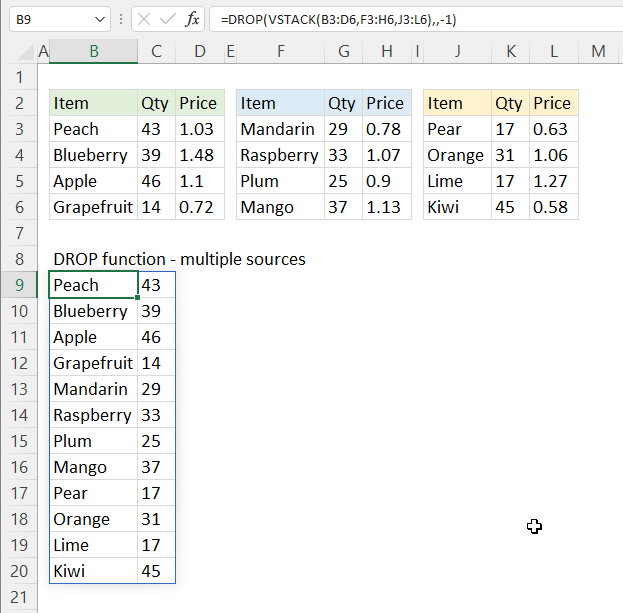The picture above shows a formula that merges three non-contiguous cell ranges and removes the last column.

Dynamic array formula in cell B9:

=DROP(VSTACK(B3:D6, F3:H6, J3:L6), , -)

### 9.1 Explaining formula

#### Step 1 - Stack values horizontally

The VSTACK function lets you combine cell ranges or arrays, it joins data to the first blank cell at the bottom of a cell range or array (vertical stacking)

VSTACK(array1, [array2],...)

VSTACK(B3:D6, F3:H6, J3:L6)

becomes

VSTACK({"Peach", 43, 1.03;"Blueberry", 39, 1.48;"Apple", 46, 1.1;"Grapefruit", 14, 0.72}, {"Mandarin", 29, 0.78;"Raspberry", 33, 1.07;"Plum", 25, 0.9;"Mango", 37, 1.13}, {"Pear", 17, 0.63;"Orange", 31, 1.06;"Lime", 17, 1.27;"Kiwi", 45, 0.58})

and returns

{"Peach", 43, 1.03;
"Blueberry", 39, 1.48;
"Apple", 46, 1.1;
"Grapefruit", 14, 0.72;
"Mandarin", 29, 0.78;
"Raspberry", 33, 1.07;
"Plum", 25, 0.9;
"Mango", 37, 1.13;
"Pear", 17, 0.63;
"Orange", 31, 1.06;
"Lime", 17, 1.27;
"Kiwi", 45, 0.58}

#### Step 2 - Remove last column

DROP(VSTACK(B3:D6, F3:H6, J3:L6), , 2)

becomes

DROP({"Peach", 43, 1.03;
"Blueberry", 39, 1.48;
"Apple", 46, 1.1;
"Grapefruit", 14, 0.72;
"Mandarin", 29, 0.78;
"Raspberry", 33, 1.07;
"Plum", 25, 0.9;
"Mango", 37, 1.13;
"Pear", 17, 0.63;
"Orange", 31, 1.06;
"Lime", 17, 1.27;
"Kiwi", 45, 0.58}, , 2)

and returns

{"Peach", 43;
"Blueberry", 39;
"Apple", 46;
"Grapefruit", 14;
"Mandarin", 29;
"Raspberry", 33;
"Plum", 25;
"Mango", 37;
"Pear", 17;
"Orange", 31;
"Lime", 17;
"Kiwi", 45}